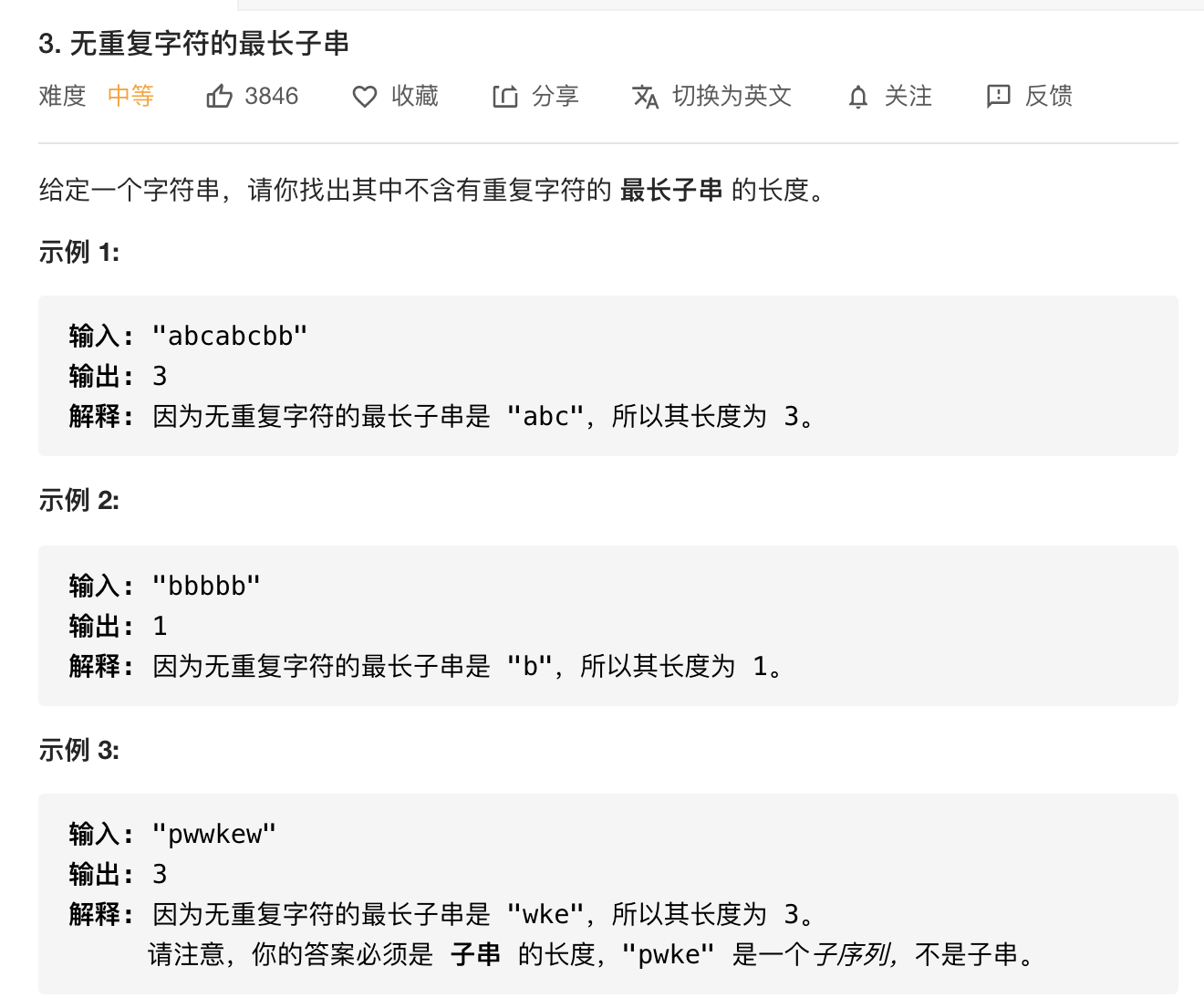# 1.题目：# 3.滑动窗口的概念：

• 利用 双指针 来实现；即左指针 表示窗口左边界，右指针 表示窗口右边界；
• 然后滑动，即意味着 有序地，左右指针移动

# 5.代码：

## HashMap（Key， Value）

• 标签：滑动窗口

• 暴力解法时间复杂度较高，会达到 O(\$N^2\$)

• 故而采取滑动窗口的方法降低时间复杂度

• 定义一个 map 数据结构存储 (k, v)，其中 key 值为字符，value 值为字符位置 +1，加 1 表示从字符位置后一个才开始不重复

• 我们定义不重复子串的开始位置为 start，结束位置为 end

• 随着 end 不断遍历向后，会遇到与 [start, end] 区间内字符相同的情况，此时将字符作为 key 值，获取其 value 值，并更新 start，此时 [start, end] 区间内不存在重复字符

• 无论是否更新 start，都会更新其 map 数据结构和结果 ans。

• 时间复杂度：O(n)

• ``````public int lengthOfLongestSubstring_滑动窗口(String s){
int n = s.length(), ans = 0;
Map<Character, Integer> map = new HashMap<>();
for(int end=0,start=0; end<n; end++){
char alpha = s.charAt(end);
if(map.containsKey(alpha)){
start = Math.max(map.get(alpha), start);//这个很重要
}
/*
* if(map.containsKey(s.charAt(i)))
* j = Math.max(map.get(s.charAt(i)),j);
* 为什么有这个判断，是因为在滑动过程中会出现当前loop出现的重复元素之间的区间，
* 可能还有其他元素也是重复的。
* 如果仅仅 j =map.get(s.charAt(i))
* 则你会忽略两个重复元素之间还有的其他元素也是重复的，
* 例如 a bba ，如果没有max，则答案会是3，因为最后一个a和前一个a的间距是3，
* 不加max就考虑不到中间两个b也是重复的。）
* */
ans = Math.max(ans, end - start + 1);
map.put(s.charAt(end), end+1);
}
return ans;
}
<!--￼1-->``````
• 返回从 fromIndex 位置开始查找指定字符在字符串中第一次出现处的索引，如果此字符串中没有这样的字符，则返回 -1。

• ``````int indexOf(String str);
<!--￼2-->
``````